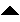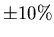Next: Sampling a time dependent Up: number6 Previous: Introduction

# Frequency Measurement

Let us first consider the problem of how to measure the frequency of a digital signal (i.e. a square wave). Signals such as this can come from a digital source or by a suitably conditioned analog source. Examples include some A/D chips, a voltage controlled oscillator, or a venerable 555 oscillator chip.

There are two basic ways of making this measurement:

• Period counting. This involves measuring the time between successive leading (or trailing) edges of the incoming pulses.
• Frequency counting. This is done by counting the number of edges that occur within a fixed time interval.
In either case one takes several measurements and averages them in order to get a useful measurement. Both methods require that the edges come in slow enough for the software to respond to their arrivals. This requirement makes the high level code presented here (listing 1) for illustration, of limited direct usefulness. To get a higher maximum frequency in a real system the edge detection would be done in assembler and/or as an ISR. (Notice that the period counter changes the hardware timer to run at 1.1MHz instead of the normal 18.2 Hz so that we get a reasonable resolution. This messing around with the hardware timer is pretty system dependent; I never got it to work from a DOS shell within Windows).

In addition, each technique has its own particular weaknesses. With period counting, there is the problem of what happens when there are missing edges. To illustrate, suppose the input signal was a 1 kHz square wave, so the time between leading edges is 1 millisecond. Given the normal vagueries of the measurement, we might expect to see a variation of, say,, so the individual measurements might vary from 0.9 to 1.1 milliseconds. The averaging of several measurements will handle this and give us an estimate of 1 millisecond with a reasonable degree of confidence. But now suppose that every once in a while we miss an edge, each time this happens we get a value of 2 milliseconds to fold into our average! This can significantly shift our estimated frequency, plus it will have a strong effect on the degree of confidence of our measurement. An occasional extra pulse can also cause problems by giving us two time values that are too short. Averaging over a large number of samples helps with this, but this might not be practical for the application. Another possibility is to sample adaptively. Adaptive requires calculating a running mean and variance, the sampling is stopped when the variance has dropped below some acceptance threshold. The problem with adaptive sampling is that it will consume significant computing resources in between each sample. A practical period sampling routine will also have a timeout provision, otherwise it will wait forever for an edge that never happens if the signal stops or is interrupted.

Frequency counting is not as adversly effected by an occasional missing or extra edge. However, frequency counting is vulnerable to counter overrun. Even if the software can keep up with the edges coming in, if the sample time is too long the counter that is accumulating the edges could overrun. A short sample time helps this since it increases the frequency at which an overrun will occur. But if the sample interval is too small, then the measurement has a reduced degree of confidence. One could also detect the overrun and handle it in some way (setting an overrun flag, stopping the count, etc.)Next: Sampling a time dependent Up: number6 Previous: Introduction
Skip Carter 2008-08-20